Technical Article

# An Intro to Pulse-width Modulation for Control in Power Electronics

September 13, 2022 by Simon Mugo

## Here you will learn all about pulse-width modulation for control in power electronics, from an introduction of definition to the application, advantages, and disadvantages of PWMs.

When it comes to power electronics, PWM (Pulse-Width Modulation) is the engine for control and has been used as the key factor in modern semiconductor electromechanical and electrical device drivers. Most power electronic system circuits are driven and controlled by PWM signals, which exist in various forms. PWM generates analog signals from a given sample of digital equipment such as microcontrollers.

In this article, we are going to have a look at what PWM is, how we can generate PWM in various ways, and its advantages, disadvantages, and applications.

### Defining Pulse-width Modulation

PWM is where an analog circuit is controlled with a microcontroller or a microprocessor digital output. While employing this technique, digital-to-analog conversion is unnecessary as the effects of noise on the signals are minimal since the signal is digital. Here the energy is input through a series of well-designed pulses rather than the use of the continuous analog or varying signal. With energy flow to the load, for example, the motor is controlled by increasing or reducing the pulse width.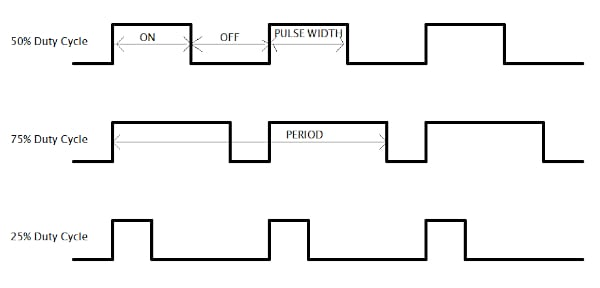##### Figure 1. PWM Waveforms for 25%, 50%, and 75% Duty Cycles. Image used courtesy of Simon Munyua Mugo

From the Figure above, the time proportion in which the pulse is high is referred to as Duty Cycle, and it has to be in percentage. The Duty Cycle is calculated based on the given equation:

$Duty\,Cycle=\frac{Pulse\,ON\,time}{Pulse\,period}\times100\%$

$Duty\,Cycle=\frac{T_{ON}}{T_{ON}+T_{OFF}}\times100\%$

$Period=T_{ON}+T_{OFF}$

To attain the average voltage, you must vary the positive pulse width. The time when the output is ON modulation will lead us to average voltage modification. All the average values are dependent on the duty cycle. The less the ON time, the smaller the average value, and the higher the ON time, the bigger the average value.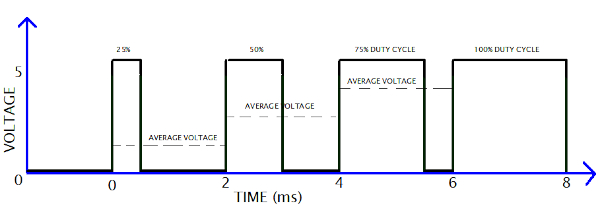### PWM Techniques

In power electronics, there exist three PWM techniques:

• Lead Edge Modulation: here the signal lead edge remains fixed while the modulation is done on the trailing edge.
• Trail Edge Modulation: here, we modulate the lead edge while we fix the trailing edge.
• Phase Correct PMW/Pulse Center Two Edge Modulation: here we fix the pulse center while we carry out modulation on both pulse edges.

For a better understanding, see the diagram below.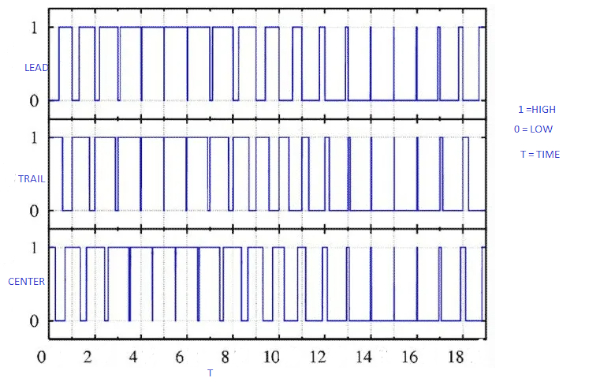### How to Generate PWM Signals

The easiest way to generate a Pulse-Width Modulation signal is by use of a comparator, as shown in Figure 4 below. The modulating signal is fed into one input of the comparator, and the other comparator input is fed with the sawtooth or non-sinusoidal wave. The comparator serves the purpose of comparing the two input signals and outputs a PWM signal.

When the sawtooth triangle signal is higher than the modulation signal, the PWM signal at the output is at a HIGH. If the sawtooth triangle signal is lower than the modulation signal, then the PWM signal at the output is LOW. Therefore, the comparator output is determined by the input signal magnitude and goes a long way in determining the width of the generated pulse at the output.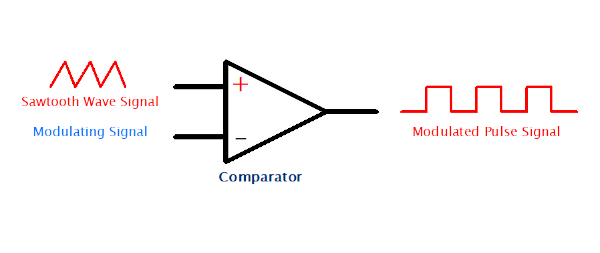### PWM Generation Using 555 Timer

The 555 timer IC is an important and versatile device in the area of power electronics. Apart from offering the ability to be configured into many helpful circuits, it also offers the ability to generate useful PWM pulses that can be obtained depending on the circuit requirements.

We have two ways in which the 555 timer IC can be used to generate the said PWM pulses. The first method involves the use of a single 555 IC and some other associated components such as the potentiometer, diodes, and capacitors. The second and last method is by involving a standard monostable 555 IC, which shall make use of an externally modulated signal.

Let’s dive deeper into the two methods.

### 555 Timer IC PWM Generation Using Diodes

This is simple and makes use of the circuit in Figure 5 below.##### Figure 5. 555 Timer IC PWM Using Diodes. Image used courtesy of Simon Munyua Mugo

The working principle behind the circuit above is way too simple. The ON time of the system is determined by the time taken by the system capacitor to charge at the two-third VCC level via pin 7 resistor, while the OFF duration is determined by the capacitor discharging time to below a third VCC via the same pin 7.

In the circuit, we can independently set or fix the charging and discharging time through the potentiometer and the used bifurcating diodes.

From the circuit in Figure 5, we have the left and the right diode. The left diode has the cathode terminal connected with 555 IC pin 7 and is used to separate the OFF time. The right diode has its anode connected to the 555 timer IC pin 7, and its function is to separate the ON time of the 555 IC output.

If the potentiometer adjustment arm tilts more toward the left side, it decreases the discharge time due to decreased resistance across the capacitor discharge path. The effect of this is increased ON time and decreased OFF duration of the 555 IC PWM.

On the other hand, when the potentiometer slider moves more on the right side, it decreases the ON time as it lowers the capacitor’s charging path resistance. The resultant effect is that we have an increased OFF time and decreased ON time of the output PWM of the 555.

### External Modulation 555 IC PWM Generation

This method is a bit more complicated than the first method discussed above. We shall introduce a varying DC on the 555 IC pin 5 to implement a proportionately varying IC output pulse width.

The circuit configuration is shown in Figure 6 below.##### Figure 6. External Modulation 555 IC PWM Generation. Image used courtesy of Simon Munyua Mugo

The wiring circuit above is known as a monostable and multivibrator mode. In this mode, the 555 IC can produce a positive pulse from pin 3 in reaction to any negative trigger at pin 2. The pulse coming from pin 3 last for a specified time which is determined by resistor RA and capacitor C1 values. Note that pin 2 is the clock input while pin 5 is the modulation input. Our output is fetched from the usual pin 3 of the IC.

The system function when a square wave pulse is fed at pin 2 determines the output frequency. A variable voltage is also input at pin 5, which voltage level determines the pulse width measured at the output point.

Pulses produced at pin 2 generate alternating triangular waves at IC pin 6 and pin 7, and its width is decided by the capacitor C1 and resistor RA. The triangular wave is compared with the voltage measured instantaneously at pin 5 for dimensioning of the pin 3 output PWMs pulses.

Let us take an example where we apply a 50HZ square wave at IC pin 2 and a voltage supply of 12V at pin 5. We should expect an output with a frequency of 50Hz and an RMS of 12V.

To reduce the RMS, we need to reduce the voltage input at pin 5. Varying this voltage varies the PWM, which will have varying RMS values.

The output waveform will be as shown in Figure 7 below.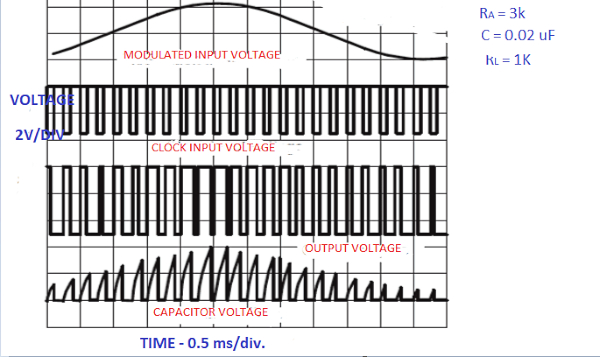##### Figure 7. The Output Waveform of PWM. Image used courtesy of Simon Munyua Mugo

• From Figure 7 above, at the top is the pin 5 modulated input voltage. The rising in the waveform is represented by the bulge in the curve of the waveform.
• The Second waveform is the clock input voltage at pin 2 of the IC. It acts as an enabler of the IC to have the ability to switch at a given frequency. The absence of this clock input means the IC cannot have the ability to work as a PWM generator.
• The third waveform is the actual Pulse-Width Modulation generator output from pin 3. From the outcome, it is obvious that the pulse width is directly proportional to the modulation signal at the top.

### Application of PWMs

PWM applications include:

• Encoding functions in telecommunications
• Audio and video amplifiers
• Smart lighting systems to control the brightness and speed of motors
• Computer motherboards to control the heat being output by the board

• Protects LEDs against overheating at a constant brightness
• Low initial cost
• Offers a high input power factor
• Enables generation of high torque by motors even when operating at low speeds
• Provides quick response time and high accuracy

• Could induce radio frequency interference

• PWM stands for Pulse-Width Modulation.
• PWM is a way of controlling analog circuits using digital outputs, preferably from microprocessors
• There exist three types of PWM techniques: lead edge, trail edge, and phase correction
• The easiest way to generate PWMs is by using a comparator
• Timer 555 IC can be used to generate PWMs through two methods: 555 Timer IC PWM generation using diodes and external modulation 555 IC PWM generator

Featured image used courtesy of Adobe Stock